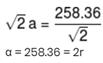Courses

# JEE Main Chemistry Mock - 3

## 25 Questions MCQ Test Mock Test Series for JEE Main & Advanced 2021 | JEE Main Chemistry Mock - 3

Description
This mock test of JEE Main Chemistry Mock - 3 for JEE helps you for every JEE entrance exam. This contains 25 Multiple Choice Questions for JEE JEE Main Chemistry Mock - 3 (mcq) to study with solutions a complete question bank. The solved questions answers in this JEE Main Chemistry Mock - 3 quiz give you a good mix of easy questions and tough questions. JEE students definitely take this JEE Main Chemistry Mock - 3 exercise for a better result in the exam. You can find other JEE Main Chemistry Mock - 3 extra questions, long questions & short questions for JEE on EduRev as well by searching above.
QUESTION: 1

Solution:
QUESTION: 2

Solution:
QUESTION: 3

### The spectrum produced due to transition of an electron from M to L shell is

Solution:
QUESTION: 4

The correct order of increasing energy of atomic orbitals is

Solution:
QUESTION: 5

Combination of two AO's lead to the formation of

Solution: According to Linear Combination Of Atomic Orbitals(LCAO), the combination of 2 AO', give 2 MO's one of which is bonding MO while the other is antibonding MO.
QUESTION: 6

Elimination of bromine from 2- bromobutane results in the formation of

Solution:
QUESTION: 7

IUPAC name of CH₃-O-C₂H₅ is

Solution:
QUESTION: 8

The name of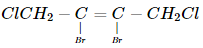according to IUPAC nomenclature system is

Solution:
QUESTION: 9

The bond order in NO is 2.5 while that in NO+ is 3. Which of the following statements is true for these two species?

Solution:

Higher the bond order, shorter will be the bond length. Thus, NO+ is having higher bond order than that of NO so NO+ has shorter bond length.

QUESTION: 10

The segment of DNA which acts as the instructional manual for the synthesis of the protein is

Solution:

A gene is the molecular unit of heredity of a living organism. It is widely accepted by the scientific community as a name given to some stretches ofdeoxyribonucleic acids (DNA) and ribonucleic acids (RNA) that code for apolypeptide or for an RNA chain that has a function in the organism, though there still are controversies about what plays the role of the genetic material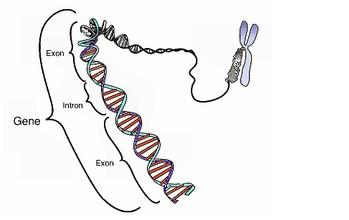QUESTION: 11

K₄[Fe(CN)₆] is a

Solution:
QUESTION: 12

What are the products formed in the reaction of xenon hexafluoride with silicon dioxide

Solution:
QUESTION: 13

The hardness of transition elements is due to their

Solution:

Transition elements show metallic character as they have low ionization energies and have several vacant orbitals in their outermost shell. This property favors the formation of metallic bonds in the transition metals and so they exhibit typical metallic properties.These metals are hard which indicates the presence of covalent bonds. This happens because transition metals have unpaired d-electrons. The d-orbital which contains the unpaired electrons may overlap and form covalent bonds. Higher the number of unpaired electrons present in the transition metals, more is the number of covalent bonds formed by them. This further increases the hardness of the metal and its strength.

QUESTION: 14

Sodium nitroprusside reacts with sulphide ion to give a purple colour due to the formation of :

Solution:
QUESTION: 15

To a solution containing equimolar mixture of sodium acetate and acetic acid, some more amount of sodium acetate solution is added. The pH of mixture solution

Solution:
QUESTION: 16

The reason for geometrical isomerism by 2-butene is

Solution:
QUESTION: 17

If chloroform is left open in air in presence of sun rays

Solution:
QUESTION: 18

Ionisation potential of hydrogen is

Solution:

Hydrogen has higher ionisation potential than chlorine, because hydrogen has only one electron and small size. This electron experiences the direct attraction of the nucleus and there is no shielding effect. Whereas in Cl the combined effect of the increase in the atomic size and the screening effect more than compensates the effect of the increased nuclear charge. Consequently, the valence electrons become less firmly held by the nucleus and ionisation potential decreases.

QUESTION: 19

Which is an example of thermosetting polymer?

Solution:
QUESTION: 20

KO2 (potassium super oxide) is used in oxygen cylinders in space and submarines because it

Solution:

Because it absorbs CO2 and increases O2 concentration according to the following reaction

4KO2 + 2CO2 → 2K2CO3 + 3O2

*Answer can only contain numeric values
QUESTION: 21

If  x = Group number of Europium (atomic number 63)
y = Period number of Americium (atomic number 95)
z = Most stable oxidation state shown by Gadolinium (atomic number 64)
then find the value of x + y + z?

Solution:
*Answer can only contain numeric values
QUESTION: 22

The decomposition of hydrogen peroxide follows first order kinetics.
2H2O2(aq) → 2H2O(ℓ) + O2(g)
If the volume of O2 gas liberated at STP in first 20 minutes of the start of decomposition is 25.00 ml, what should be the total volume of O2 gas (in ml) collected in time, t >> t1/2.
(t1/2 for the decomposition of H2O2 is 10 min)

Solution: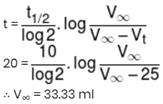*Answer can only contain numeric values
QUESTION: 23

How many H-atom can be exchanged by D-atom when the following compound is kept in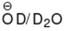solution for long time?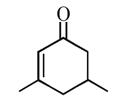Solution:
*Answer can only contain numeric values
QUESTION: 24

Find out the number of following orders which are INCORRECT against the mentioned properties :
(i) Ortho hydrogen > Para hydrogen (Stability at low temperature)
(ii) Ethanol > Glycerol (Viscosity)
(iii) D2 < He (Boiling Point)
(iv) HF > H2O (Melting point)
(v)  H3BO3 > BF3 (Melting point)
(vi) NH3 < SbH3 (Boiling point)
(vii) H2O2 > H2O (Strength of hydrogen bond)
(viii) H2O < D2O (Freezing point)
(ix)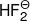< HF (Strength of hydrogen bond)
(x) D2O < H2O (Bond energy)

Solution:
*Answer can only contain numeric values
QUESTION: 25

A metal crystallises as simple cubic crystal. The distance between the next nearest neighbours of each atom ispm. The atomic radius of metal atoms (in pm) is

Solution: